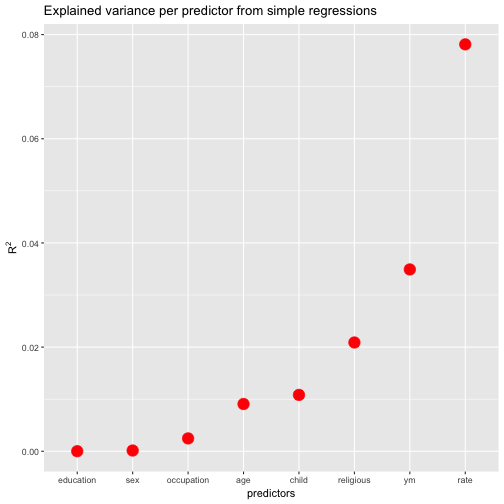# EDIT: Running multiple simple regressions with purrr

## September 26, 2016

Reading time ~8 minutes

EDIT based on comments/ suggeestions from @JonoCarroll Disqus profile and @tjmahr twitter profile. See below (last step; look for “EDIT”).

Thanks for the input!reading time: 10 min.

Hadley Wickham’s purrr has given a new look at handling data structures to the typical R user (some reasoning suggests that average users doesn’t exist, but that’s a different story).

I just tried the following with purrr:

• Meditate about the running a simple regression, FWIW
• Take a dataframe with candidate predictors and an outcome
• Throw one predictor at a time into the regression, where the outcome variable remains the same (i.,e multiple simple regressions (one predictor) where the predictor is changed at each run but the outcome remains the same)
• tidy up the resulting $R^2$ in some nice format

I found that purrr does the job nicely, and it’s quite instructive to see purrrat work, I think. That’s why I wrote it up in this short post:

library(purrr)
library(ggplot2)
library(dplyr)
library(broom)
library(knitr)  # for kable
data(Fair, package = "Ecdat") # extramarital affairs dataset
glimpse(Fair)

## Observations: 601
## Variables: 9
## $sex <fctr> male, female, female, male, male, female, female, ... ##$ age        <dbl> 37, 27, 32, 57, 22, 32, 22, 57, 32, 22, 37, 27, 47,...
## $ym <dbl> 10.00, 4.00, 15.00, 15.00, 0.75, 1.50, 0.75, 15.00,... ##$ child      <fctr> no, no, yes, yes, no, no, no, yes, yes, no, yes, y...
## $religious <int> 3, 4, 1, 5, 2, 2, 2, 2, 4, 4, 2, 4, 5, 2, 4, 1, 2, ... ##$ education  <dbl> 18, 14, 12, 18, 17, 17, 12, 14, 16, 14, 20, 18, 17,...
## $occupation <int> 7, 6, 1, 6, 6, 5, 1, 4, 1, 4, 7, 6, 6, 5, 5, 5, 4, ... ##$ rate       <int> 4, 4, 4, 5, 3, 5, 3, 4, 2, 5, 2, 4, 4, 4, 4, 5, 3, ...
## $nbaffairs <dbl> 0, 0, 0, 0, 0, 0, 0, 0, 0, 0, 0, 0, 0, 0, 0, 0, 0, ...  Fair %>% dplyr::select(-nbaffairs) %>% # exclude outcome, leave only predictors map(~lm(Fair$nbaffairs ~ .x, data = Fair)) %>%
map(summary) %>%
map_dbl("r.squared") %>%
tidy %>%
dplyr::arrange(desc(x)) %>%
rename(r.squared = x) -> r2s

kable(r2s)

names r.squared
rate 0.0781272
ym 0.0349098
religious 0.0208806
child 0.0108181
age 0.0090701
occupation 0.0024613
sex 0.0001377
education 0.0000059

Ok, that appears to be the list of the $R^2$ for each simple (one-predictor) regression we have run.

Let’s do a quick sense check with the standard way:

lm1 <- lm(nbaffairs ~ rate, data = Fair)

summary(lm1)

##
## Call:
## lm(formula = nbaffairs ~ rate, data = Fair)
##
## Residuals:
##     Min      1Q  Median      3Q     Max
## -3.9063 -1.3989 -0.5631 -0.5631 11.4369
##
## Coefficients:
##             Estimate Std. Error t value Pr(>|t|)
## (Intercept)   4.7421     0.4790   9.900   <2e-16 ***
## rate         -0.8358     0.1173  -7.125    3e-12 ***
## ---
## Signif. codes:  0 '***' 0.001 '**' 0.01 '*' 0.05 '.' 0.1 ' ' 1
##
## Residual standard error: 3.17 on 599 degrees of freedom
## Multiple R-squared:  0.07813,	Adjusted R-squared:  0.07659
## F-statistic: 50.76 on 1 and 599 DF,  p-value: 3.002e-12

summary(lm1)$r.squared  ##  0.07812718  summary(lm1)$coefficients[2, 4]  #p.value

##  3.002385e-12


Seems to work. To get details of the object summary(lm1), use str(summary(lm1)).

How many did we run? Just the number of columns minus one (the outcome variable).

ncol(Fair)-1

##  8


FWIW, let’s plot the resulting values (and sort the predictors by descending values).

ggplot(r2s, aes(x = reorder(names, r.squared), y = r.squared)) +
geom_point(size = 5, color = "red") +
ylab(expression(R^{2})) +
xlab("predictors") +
ggtitle("Explained variance per predictor from simple regressions")Wait, one more thing. Suppose we are not only interested in $R^2$, but in the p-values (OMG). How to get both values from purrr?.

EDIT (the following part has changed)

Thanks for comments from @JonoCarroll Disqus profile and @tjmahr twitter profile, the last step - extracting the p-values - is now changed, and I think improved.

Fair %>%
dplyr::select(-nbaffairs) %>%  # exclude outcome, leave only predictors
map(~lm(Fair$nbaffairs ~ .x, data = Fair)) %>% map(summary) %>% map(broom::tidy) %>% map_df("p.value") %>% round(3) %>% mutate(variable = c("intercept", "predictor")) -> ps  library(htmlTable) htmlTable(ps)  sex age ym child religious education occupation rate variable 1 0 0.465 0.019 0 0 0.1 0.002 0 intercept 2 0.774 0.02 0 0.011 0 0.952 0.225 0 predictor So what I did above basically is: • Run a linear model on each predictor • Get a summary of each model • Tidy (with broom) each summary • Get the sublist (column) p.value from each list (model), and save the result as a data frame To get a whole bunch of relevant statistics, you can use glance: Fair %>% dplyr::select(-nbaffairs) %>% # exclude outcome, leave only predictors map( ~lm(Fair$nbaffairs ~ .x, data = Fair)) %>%
map(summary) %>%
map_df(glance) %>%
round(3)

##   r.squared adj.r.squared sigma statistic p.value df
## 1     0.000        -0.002 3.301     0.083   0.774  2
## 2     0.009         0.007 3.287     5.483   0.020  2
## 3     0.035         0.033 3.243    21.667   0.000  2
## 4     0.011         0.009 3.284     6.551   0.011  2
## 5     0.021         0.019 3.267    12.774   0.000  2
## 6     0.000        -0.002 3.302     0.004   0.952  2
## 7     0.002         0.001 3.297     1.478   0.225  2
## 8     0.078         0.077 3.170    50.764   0.000  2


Thanks for comments from @JonoCarroll Disqus profile and @tjmahr twitter profile, the last step - extracting the p-values - is now changed, and I think improved.

### This blog has moved

This blog has moved to Adios, Jekyll. Hello, Blogdown!… Continue reading

#### Wie gut schätzt eine Stichprobe die Grundgesamtheit?

Published on November 17, 2017

#### Some thoughts on tidyveal and environments in R

Published on November 16, 2017